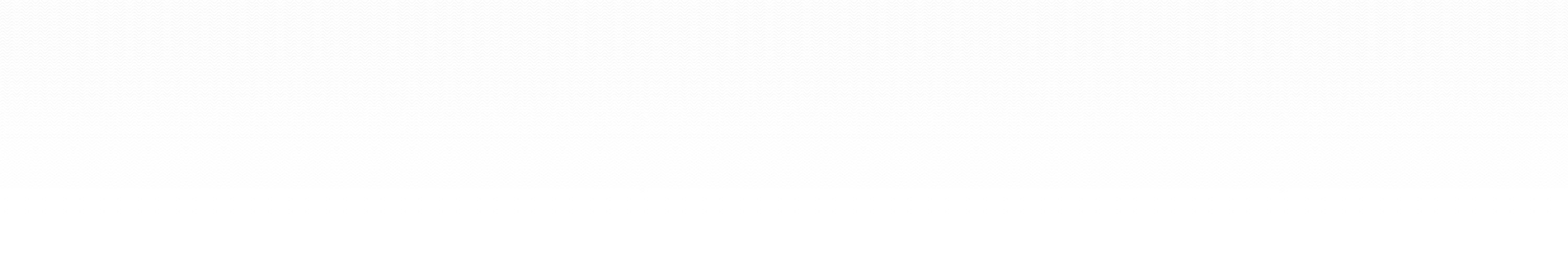### Maple code (older worksheets)

Right-click (Windows) or ctrl-click (Mac) to download the files to your machine.

Code to generate plots of the stability region of various one-step methods in the complex plane [pdf | Winter 2011]
Illustrations of what goes wrong when you try to use an unstable numerical scheme [pdf | Winter 2011]
Stability properties of various one-step methods
Derivation and error analysis of the explicit multi-step Adams-Bashford stencils [pdf | Winter 2011]
Some examples of how to use pdsolve
Derivation of finite difference representations of higher derivatives [pdf | Winter 2011]
Presentation of a forward-time centered-space solver for the diffusion equation [pdf | Winter 2011]
Illustration of how to code the FTCS stencil for the diffusion equation
An introduction of the BTCS and Crank-Nicholson stencils as well as the associated von Nuemann stability analysis [pdf | Winter 2011]
How to use the Crank-Nicolson method to solve a nonlinear parabolic PDE [pdf | Winter 2011]
How to solve the ODE boundary value problem using the shooting method [pdf | Winter 2011]
How to solve the linear ODE boundary value problem using matrix methods [pdf | Winter 2011]
How to solve the nonlinear ODE boundary value problem using relaxation matrix methods (i.e. Newton's method) [pdf | Winter 2011]
Presentation of 5-point and 9-point finite difference stencils for the Laplacian in two dimensions [pdf | Winter 2011]
Solution of Laplace's equation on the unit square with Dirichlet BCs [pdf | Winter 2011]
Solution of Poisson's equation on a rectangular region with Dirichlet BCs and an arbitrary source [pdf | Winter 2011]